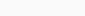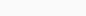# Rbse Solutions for Class 7 Maths Chapter 7 – Congruence of Triangles

Exercise 7.2 Solutions

### Access Answers to NCERT Class 7 Maths Chapter 7 – Congruence of Triangles Exercise 7.1

1. Complete the following statements:

(a) Two line segments are congruent if ___________.

Solution:-

Two line segments are congruent if they have the same length.

(b) Among two congruent angles, one has a measure of 70o; the measure of the other angle is ___________.

Solution:-

Among two congruent angles, one has a measure of 70o; the measure of the other angle is 70o.

Because, if two angles have the same measure, they are congruent. Also, if two angles are congruent, their measure are same.

(c) When we write ∠A = ∠B, we actually mean .

Solution:-

When we write ∠A = ∠B, we actually mean m ∠A = m ∠B.

2. Give any two real-life examples for congruent shapes.

Solution:-

The two real-life example for congruent shapes are,

(i) Fan feathers of same brand.

(ii) Size of chocolate in the same brand.

(iii) Size of pens in the same brand

3. If ΔABC ≅ ΔFED under the correspondence ABC ↔ FED, write all the corresponding congruent parts of the triangles.

Solution:-

Two triangles are congruent if pairs of corresponding sides and corresponding angles are equal.

All the corresponding congruent parts of the triangles are,

∠A ↔ ∠F, ∠B ↔ ∠E, ∠C ↔ ∠D

Correspondence between sides:

4. If ΔDEF ≅ ΔBCA, write the part(s) of ΔBCA that correspond to

(i) ∠E (ii)  (iii) ∠F (iv)

Solution:-

From above the figure we can say that,

The part(s) of ΔBCA that correspond to,

(i) ∠E ↔ ∠C

(ii)(iii) ∠F ↔ ∠A

(iv)### 1 thought on “Rbse Solutions for Class 7 Maths Chapter 7 – Congruence of Triangles”

Patio umbrellas sold at Costco recalled after reports of fires गर्मी में लू लगने से बचाव करेंगे यह खाद्य पदार्थ, आज ही खाना करें शुरू ट्रेन के बीच में ही AC कोच क्यों लगाए जाते हैं? Rbse books for class 1 to 12 hindi medium 2021-22